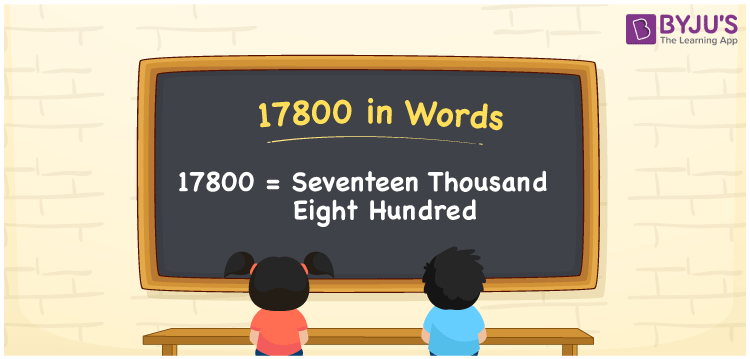# 17800 in words

17800 in words is written as Seventeen Thousand Eight Hundred. In 17800, 1 has a place value of ten thousand and 7 has a place value of thousand and 8 has a place value of hundred. The article on Place Value gives more information. The number 17800 is used in expressions that relate to money, distance, length, count and many more. For example, “Seventeen Thousand Eight Hundred centimeters is the same as 178 meters.” Another example, “For the World Environment day, an NGO planned to plant Seventeen Thousand Eight Hundred saplings.”

 17800 in words Seventeen Thousand Eight Hundred Seventeen Thousand Eight Hundred in Numbers 17800

## 17800 in English Words## How to Write 17800 in Words?

We can convert 17800 to words using a place value chart. This can be done as follows. The number 17800 has 5 digits, so let’s make a chart that shows the place value up to 5 digits.

 Ten thousand Thousands Hundreds Tens Ones 1 7 8 0 0

Thus, we can write the expanded form as:

1 × Ten thousand + 7 × Thousand + 8 × Hundred + 0 × Ten + 0 × One

= 1 × 10000 + 7 × 1000 + 8 × 100 + 0 × 10 + 0 × 1

= 17800

= Seventeen Thousand Eight Hundred.

17800 is the natural number that is succeeded by 17799 and preceded by 17801.

17800 in words – Seventeen Thousand Eight Hundred.

Is 17800 an odd number? – No.

Is 17800 an even number? – Yes.

Is 17800 a perfect square number? – No.

Is 17800 a perfect cube number? – No.

Is 17800 a prime number? – No.

Is 17800 a composite number? – Yes

## Solved Example

1. Write the number 17800 in expanded form

Solution: 1 x 10000 + 7 x 1000 + 8 x 100 + 0 x 10 + 0 x 1

Or Just 1 x 10000 + 7 x 1000 + 8 x 100

We can write 17800 =10000 + 7000 + 800 + 0 + 0

= 1 x 10000 + 7 x 1000 + 8 x 100 + 0 x 10 + 0 x 1

## Frequently Asked Questions on 17800 in words

Q1

### How to write 17800 in words?

17800 in words is written as Seventeen Thousand Eight Hundred.
Q2

### State whether True or False. 17800 is divisible by 3?

False. 17800 is not divisible by 3.
Q3

### Is 17800 divisible by 10?

Yes. 17800 is divisible by 10.